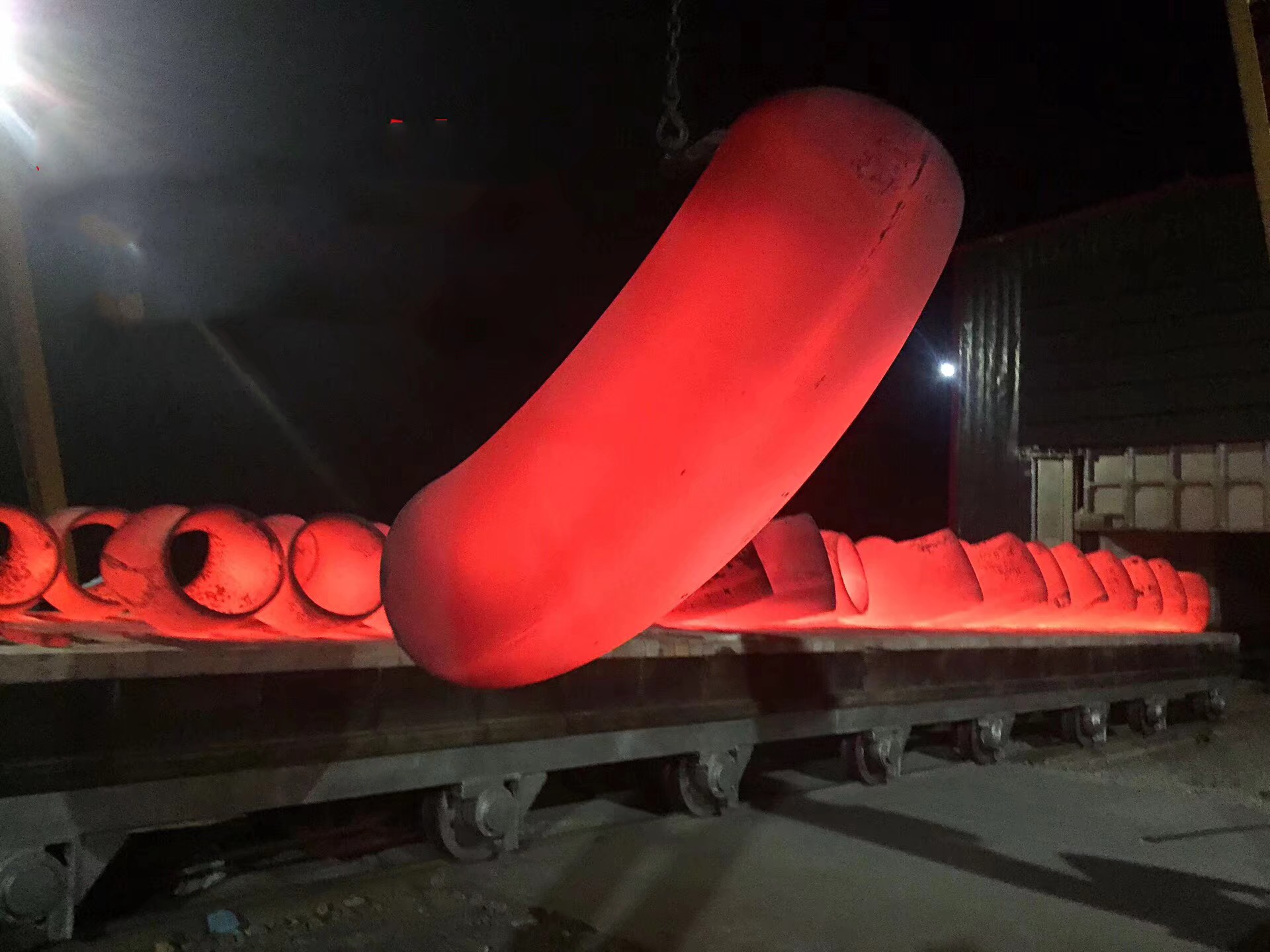Butt Weld Pipe Fittings 90 Degree A234wpb Elbow in pipelines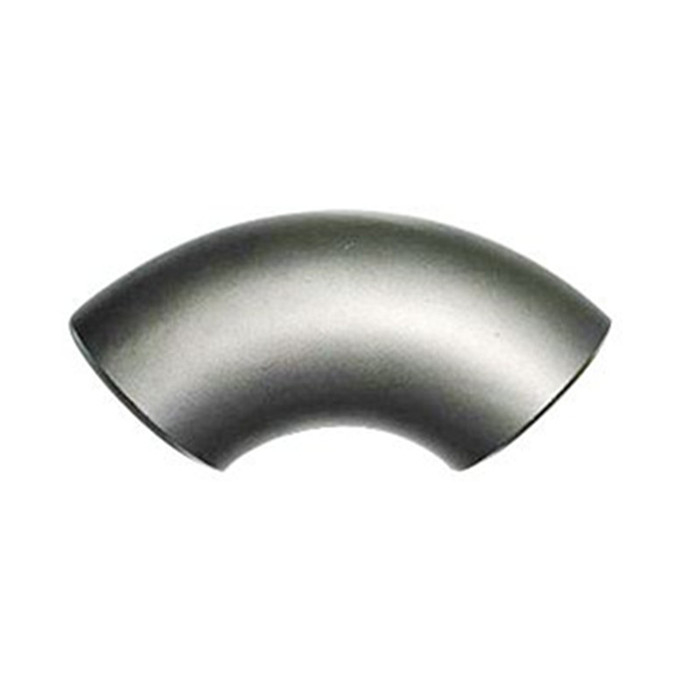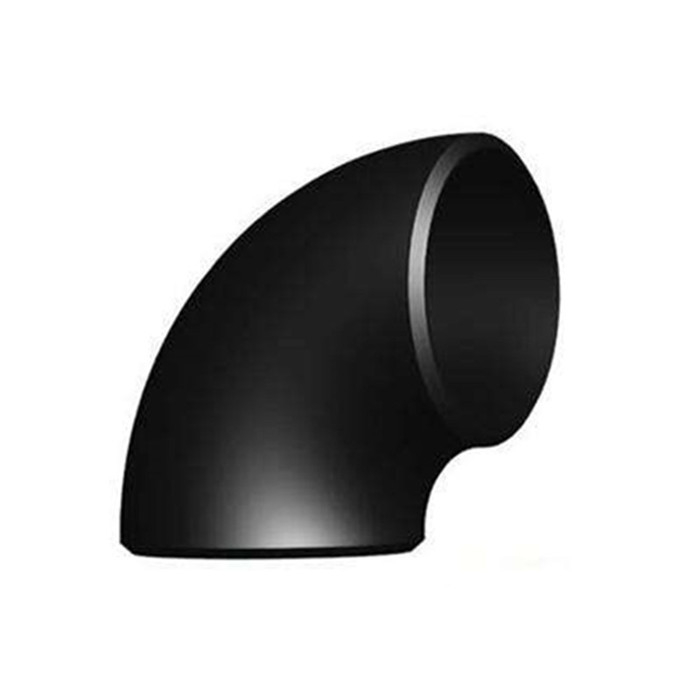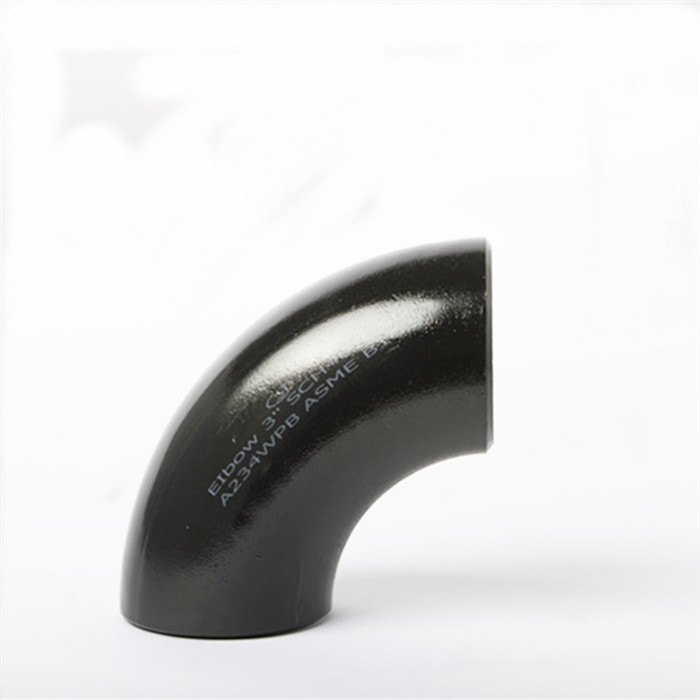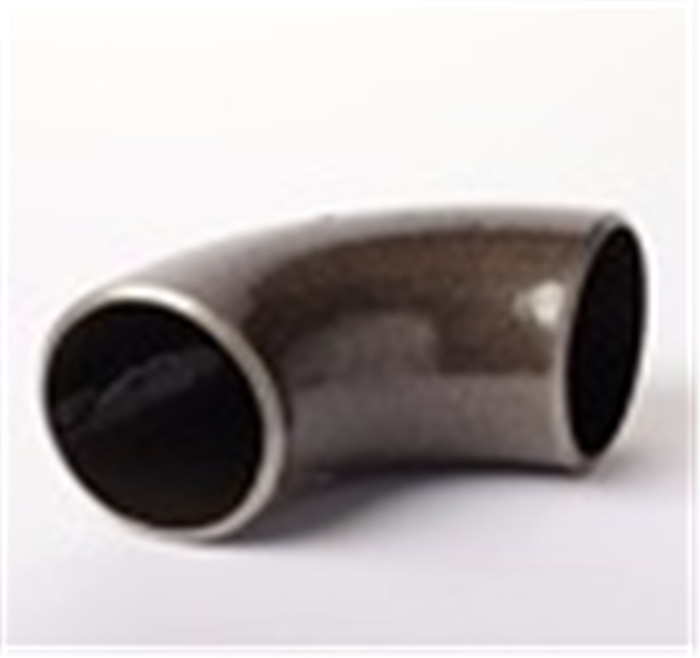Model
JS-E

## Item specifics

Size
1/2
material
CS, MS
Usage
water, oil and gas
Surface treatment
blasting, black painting, anti-rust oil
Ends
Bevel ends
Connection
welding

## Description

Butt weld Carbon steel LR SR Elbows for water supply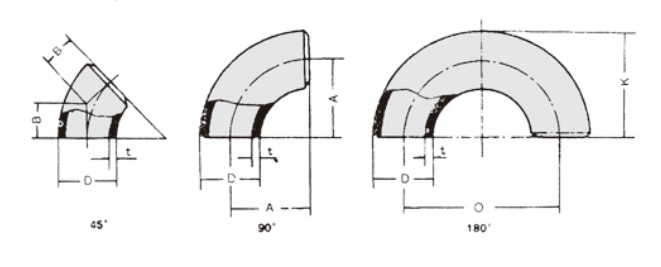How to calculate the 90 degree Elbows?

For standard degrees of steel pipe elbows such as 45° and 90°, pipe elbow center to end dimensions are available in standard pipe charts. But many times, custom elbow angles are required at site which should be cut from standard 45° or 90° elbows.

Formula for calculating center to end distance of such elbows is as follows:

Elbow length in mm = Tan(Elbow Angle/2) X Elbow Radius in mm

Where:

For 90° Long Radius elbows, center to end dimension given in dimension tables of ANSI B16.9 is same as radius of elbow. This is because Tan(90/2) i.e. Tan 45 is 1.

Normally custom steel elbow angles from 45 degree to 90 are cut from 90 degree standard elbow. But for custom elbow angles smaller than 45 degree, JS FITTINGS elbow is normally cut from existing standard 45 degree elbow. Center to end dimension given in dimension tables for 45 degree elbow must be divided by Tan(22.5) to get elbow radius for standard 45 degree elbow. Then we can use above formula to get elbow angle for custom degrees.

Same procedure applies to 3D elbows.

Example 1:

Calculate elbow center to end dimension for 4 inch nominal pipe diameter elbow at 60 degree angle, cut from 90 degree LR elbow.

From ASME B16.9, center to elbow dimension for 4 in elbow is 152 mm.

Length = Tan (60/2) X 152

Length = 0.57735027 X 152

Length = 87.757 i.e. 88 mm Approx.

### Example 2:

Calculate elbow center to end dimension for 2 inch nominal pipe diameter elbow at 30 degree angle, cut from 45 degree LR elbow.

From ASME B16.9, center to elbow dimension for 2 inch 45 degree elbow is 35 mm.

Radius of elbow = 35/0.4142 = 84.5 mm

Length = 0.26795 X 84.5

Length = 22.64 i.e. 23 mm Approx.